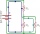Resistance

A resistor having an electrical resistance of 1.5 k ohms passes an electrical current of 0.1 A. Calculate what voltage is between the terminals of the resistor.

Result

U =  150 V

Solution:Leave us a comment of example and its solution (i.e. if it is still somewhat unclear...):Be the first to comment!Next similar examples:

1. Effective and mean voltageA voltage divider consisting of resistors R1 = 103000 Ω and R2 = 197000 Ω is connected to the ideal sine wave voltage source, R2 is connected to a voltmeter which measures the mean voltage and has an internal resistance R3 = 200300 Ω, the measured value is
2. ResistanceDetermine the resistance of the bulb with current 200 mA and is in regular lamp (230V).
3. Theorem proveWe want to prove the sentence: If the natural number n is divisible by six, then n is divisible by three. From what assumption we started?
4. Factory and divisionsThe factory consists of three auxiliary divisions total 2,406 employees. The second division has 76 employees less than 1st division and 3rd division has 212 employees more than the 2nd. How many employees has each division?
5. Volleyball8 girls wants to play volleyball against boys. On the field at one time can be six players per team. How many initial teams of this girls may trainer to choose?
6. One halfOne half of ? is: ?
7. Two equationsSolve equations (use adding and subtracting of linear equations): -4x+11y=5 6x-11y=-5
8. 6 termsFind the first six terms of the sequence. a1 = 7, an = an-1 + 6
9. PowersExpress the expression ? as the n-th power of the base 10.
10. TreesA certain species of tree grows an average of 0.5 cm per week. Write an equation for the sequence that represents the weekly height of this tree in centimeters if the measurements begin when the tree is 200 centimeters tall.
11. Linear systemSolve a set of two equations of two unknowns: 1.5x+1.2y=0.6 0.8x-0.2y=2
12. Nineteenth memberFind the nineteenth member of the arithmetic sequence: a1=33 d=5 find a19
13. Functions f,gFind g(1) if g(x) = 3x - x2 Find f(5) if f(x) = x + 1/2
14. First manWhat is the likelihood of a random event where are five men and seven women first will leave the man?
15. Hotel roomsIn the 45 rooms, there were 169 guests, some rooms were three-bedrooms and some five-bedrooms. How many rooms were?
16. Fifth of the numberThe fifth of the number is by 24 less than that number. What is the number?
17. Equations - simpleSolve system of linear equations: x-2y=6 3x+2y=4